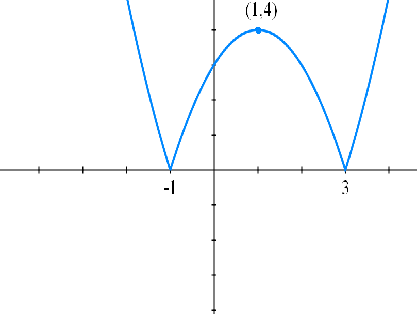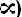# consider the equation |x^2-2x-3|=m,:m belongs to R1] values of m so that the given equation has four solution in the interval2] if the eqn has 3 soln then3]if the given eqn has two solutions then

9 years ago

Graph of |x^2-2x-3| is:Now,

1] values of m so that the given equation has four solution in the interval =

If m belongs to (0,4)

2] if the eqn has 3 soln then =

m belongs to {4}

 3] if the given eqn has two solutions then: m belongs to (4,U {0}     Plz Approve!